# Description

## Preface

The underlying flow regime (UFR) considered is the flow in a stagnation point. This type of flow regime is of considerable technical relevance since it occurs whenever a flow impinges onto a solid object, typically in turbo-machinery or the leading edge of an aerofoil. Nowadays, for the determination of the flow-field in these configurations, CFD is used and hence this URF is always a part of such calculations. However, since the flow in this region is usually turbulent the predictions depend strongly on the quality of the chosen turbulence model. It is well known that the flow in stagnation point is often incorrectly predicted by the turbulence models. Even when the stagnation point region is not of interest per se, any erroneous calculation can distort the rest of the computed flow field, for example in the case for the prediction of heat transfer in the vicinity of the stagnation point. An overproduction of turbulent kinetic energy, as is typically predicted by two-equation turbulence models, results in an overestimation of heat transfer. It is evident that this failure is of strong relevance for the design of cooled turbine blades.

The choice of the turbulence model is always a compromise between accuracy of the predicted results and the numerics (i.e. stability, convergence, CPU time). In the following the focus is on the two-equation models. This type of turbulence model is widely used because of its numerical stability and relatively simple formulation. The main disadvantage of the two-equation models is their poor performance under adverse pressure gradients, which is also an important feature of stagnation point flows. In order to overcome these deficits of the model many different modifications where proposed.

## Introduction

For most technical relevant applications the stagnation point flow is turbulent, therefore in the following only turbulent flows are considered. Whenever the fluid impinges a solid surface a stagnation point flow occurs. The fluid is decelerated in the region ahead of the stagnation point. This condition results in an adverse pressure gradient which may result in separation. Hence, it is crucial that the simulation is able to predict the onset of the separation but unfortunately most turbulence models fail to calculate correctly this key issue. Furthermore, this UFR is often the starting point of boundary layer growth. It is obvious that the correct prediction of the turbulence is of importance since the size and development of the boundary layer depends strongly on the strength of the turbulence.

Flow in stagnation region is a URF with relevance to many Application Challenges. It is of special importance for the following ACs:

 Low-Speed Centrifugal Compressor 6-02 Annular Compressor Cascade Without Clearance 6-03 Pump Turbine 6-04 Annular Compressor Cascade With Tip Clearance 6-05 Gas Turbine Nozzle Cascade 6-06 High Speed Centrifugal Compressor 6-08 High Speed Axial Compressor 6-09 Axial Compressor Cascade 6-10 Turbine Cascade With Cooling Holes 6-11 Steam Turbine Rotor Cascade 6-12

Turbulence can be regarded as a superimposition of vortices of several sizes. The largest eddies are in the order of magnitude of the dimension of the flow. On the other hand, the smallest eddies are limited in their size through the viscous forces of the fluid. The kinetic energy, hence the momentum, from the mean motion of the flow is transferred into those large eddies which subsequently feed the smaller eddies through vortex stretching. This process, the so-called energy cascade continues until the viscous effects are dominant, where the energy dissipates into heat. The rate at which the energy is extracted from the mean flow is matched to the rate of the energy transfer across the energy spectrum to small dissipating eddies ε.

The momentum transfer by the turbulent motion generates shear stresses which is a key issue for the turbulence production. The Reynolds stresses generated in this process are much larger than the viscous stress, with the exception in the vicinity of the wall, where these two stresses are comparable in magnitude.

The structures of turbulent flow are mainly determined by the origin of the turbulence in the transition process. The effect on turbulence in a stagnation point flow is considerably different than that generated by shear. In the former case a local irrotational strain field is created with stretching in lateral direction (i.e. in direction of the surface) and compression in wall-normal direction. Furthermore, another significant feature of turbulence in the stagnation region is the ratio of production of turbulent kinetic energy k to dissipation ε. In this UFR this particular ratio is much bigger than 1, in contrast to shear layers where the ratio is approximately equal to 1.

## Review of UFR studies and choice of test case

Over the years many different turbulence models with various levels of complexity were developed. Each of these models has its respective strengths and weaknesses. The decision which model should be used for the computation can be very difficult, since the chosen model will strongly influence the numerical performance and accuracy of the prediction. The selection of the turbulence model is dependent on the considered case, since no single model is superior to all other models for a wide range of flow conditions. Higher order models do not have to be the best choice due to their increased complexity in coding and possible reduced convergence behaviour. On the other hand, simpler models are usually designed for specific problems and if used for other configurations they often fail.

The first turbulence models where algebraic models which where based on the mixing length concept. This mixing length had to be specified for each case explicitly, which is often difficult to determine. As a next step in the complexity in turbulence modelling were the one-equation models. The older types of models solve one transport equation for turbulent kinetic energy with the supply from a characteristic length scale. Again, similar to the algebraic models its application range is limited. The newer approaches of the one-equation models solve a transport equation for the eddy viscosity. This approach does not need a characteristic length scale but it requires some calibration which depends on the nearest no-slip surface. Due to the fact of the need of case-specific calibrations, this model type is again somehow restricted in its use for complex technical applications.

Two-equation models are the logical extension of the former models. Nearly all two-equation models solve one equation for the turbulent kinetic energy k. The second equation can be used to solve for a variable z which has the dimension of z = kmLn; where L is a length. Depending on the choice of m and n, the variable z is either e.g. a time-scale (m: 0.5, n: -1 results in √k/L) or the dissipation ε (m: 1.5, n: -1 results in k3/2/L). The de facto standard two-equation model is the k-ε model which was used in many different applications.

Other turbulence models which were not derived from the concepts outlined above, such as LES, RANS or PDF exhibit in many cases better performances but are either very time consuming or at the moment not applicable for technical relevant cases. However, the k-ε model and the models derived thereof offer a reasonable balance between numerics and quality of the predictions despite its known deficits. Therefore, the focus lies on the two-equation models only.

Originally, the k-ε model was developed for flows at high Reynolds numbers. This model shows an overproduction of turbulent kinetic energy in the vicinity of a wall and therefore an overestimation of heat transfer. The reasons for this shortcoming of the standard k-ε-model are well documented in literature (Durbin 1996, Menter et al. 1997, Laurence 2002). It is also frequently observed that this model predicts high levels of turbulence upstream of a stagnation point, which is then transported around the body (Menter 1992, Kato and Launder 1993, Kral et al. 1996). Through this effect, the development of boundary layer is distorted and does not correspond anymore with the experimental measurements.

In the UFR considered here the flow interacts with a surface at low Reynolds numbers. In order to apply the k-ε model in the vicinity of the wall, the equations have to be integrated through the viscous sublayer which requires an augmentation of the model by low Reynolds number terms and damping factors. As another possibility one can incorporate wall functions. This boundary condition requires that the first grid node of the mesh lies outside the viscous sublayer, i.e. in the logarithmic part of the boundary layer. Obviously for complex geometries, as is common in e.g. turbomachinery, it is difficult to position the first grid nodes over the surface, since the thickness of the viscous sublayer is not known beforehand. Furthermore, the k-ε model underestimates the effects of swirl and longitudinal streamline curvatures. It is also of significance for the stagnation point flow that this model does not work well for boundary layers in adverse pressure gradients. To overcome these deficits series of proposals have been presented in the past, where these modifications can be grouped under several modification strategies which are summarised below.

### Turbulent Kinetic Energy Modifications

The governing equations for the turbulent kinetic energy k and the dissipation ε have both the same structure. The equation for k is stated as: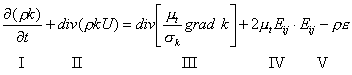and by replacing k by ε and multiplying the respective terms (IV) by C·ε/k and (V) by C·ε/k one obtains the equation for ε. Each term of these equations represents the following:

I) Rate of change of k or ε

II) Convectional transport of k or ε

III) Diffusional transport of k or ε

IV) Production rate of k or ε

V) Destruction rate of k or ε

In order to complete the standard k-ε model 5 constants are needed, which are determined through data fitting. In this model is the turbulent transport based on the gradient diffusion. Furthermore, with the Prandtl number are the diffusivities of ε and k, respectively, connected to the eddy viscosity.

The production rate of k, or also turbulent energy, is the work done by the mean strain rate against the turbulent stresses. It can also be expressed as: -uv × (∂U/∂y) = (shear stress) × (mean velocity gradient). The destruction rate on the other hand, is equal to ε/T, where T is the turbulent time scale which is proportional to k/ε and ε is the rate at which the turbulent energy is dissipated by viscosity.

A feature of the k-ε model is that this model predicts too large turbulent energy levels in the areas with large rates of strain — which is typically in stagnation point flows. Therefore, the problem is not the stagnation point per se, but the large strain rates which prevail under these conditions. In the literature many proposals can be found to overcome these problems.

One possibility to solve the stagnation point anomaly is to modify the production term in the kinetic energy equation (e.g. Kato and Launder (1993), Menter (1992), Menter et al. (1997), Strahle (1985)). Menter et al. (1997) showed that in a boundary layer type flow with the dominant strain rate S = ∂U/∂x the production term is: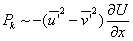whereas the formulation of Pk in the k-ε model results in: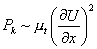The exact formulation of the dominant strain rate is linear and can even change sign. However in the two-equation model is this not the case. The quadratic term increases much stronger, regardless if the flow is accelerated, or in the UFR considered here, decelerated. This quadratic term can be replaced by |S|·|Ω|, where |S| is the strain rate as defined above and |Ω| is the vorticity tensor. With this formulation the production term vanishes in irrotational flows and hence overcomes the problematic of the stagnation point flow. However, physically it is not correct that the production term Pk is equal to zero in irrotational flows.

In a similar way Kato and Launder (1993) modified Pk while Menter (1992) substituted the strain rate with the vorticity in the production term in the following way: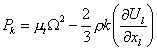However, Durbin (1996) points out, that this modification would cause excessive production in rotating or swirling flows.

Instead of introducing new terms in the production term of the turbulent kinetic energy another approach is often proposed. Based on the observation that in the UFR the ratio of production of k to dissipation ε can have very high levels, a possibility is to limit the growth of the production term. On the other hand, this ratio is approximately equal to one in free shear layers. This solution method does not require special treatment of the formulae in cases of rotating flows, free shear layers or for solid body rotation. Menter (1993) and Kral et. al (1996) used for their respective cases limiters, where in the former case it is formulated as: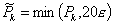By using a factor of 20 is very conservative, since the ratio of production to dissipation hardly reaches such large values.

### Dissipation Modifications

Turbulent kinetic energy is dissipated by the work of the smallest eddies against the viscous stresses of the fluid. The dissipation ε is equal to the rate at which the turbulent kinetic energy is removed from the energy containing eddies (Malalasekera & Versteeg, 1996). This is always the main destruction term in the turbulent kinetic energy equation. However, the exact equation for the dissipation ε includes many unknown and un-measurable terms which increase the difficulties of modelling ε.

Rodi and Scheurer (1986) pointed out the special role of irrotational straining of the fluid in the process of energy transport to the small dissipating eddies. The generation term of the ε-equation includes rotational and irrotational strain rates. If the later strain rate is multiplied by a larger empirical coefficient than for the former strain rate, then the dissipation increases (Rodi and Scheurer, 1986). However, this proposal includes again an empirical coefficient. Similarly, Yakhot et al. (1992) also proposed to modify the dissipation equation in order to increase the dissipation, but Laurence (2002) came to the following conclusion: Although the model of Yakhot et al. (1992) results in lower levels of turbulence, it is the production of k which is erroneous (i.e. overestimated) by the eddy viscosity models, and not the level of ε.

Wilcox (1993) suggested replacing ε by the vorticity ω. An important feature of this model is, that there is no need for damping functions in the near wall region. The disadvantage of this model is its sensitivity towards the freestream values of ω (Køllgaard Voigt, 2000). However, based on this model a few derivative models were proposed (e.g. Menter, 1994).

### Time Scale Bound

Durbin (1996) found that the turbulent time scale T in the transport equation for ε becomes very large as the stagnation point is approached. When T, which is equal to k/ε, is increased then the dissipation rate decreases, which leads to higher levels of kinetic energies. As a consequence of this observation, Medic and Durbin (2002) proposed a T bound in the form of: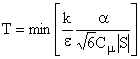This T bound was derived under the assumption of non-negative eigenvalues of the Reynolds stress tensor. This means that the non-negative eigenvalues ensure that the Reynolds stresses, which are the entity in the Navier Stokes equations which are responsible for the transport of energy from the turbulence to the mean flow, are positive. This constraint ensures that the model is physically realistic. Furthermore, this limiter is applied only to the high Reynolds number region. For the model proposed by Medic and Durbin (2002) a standard k-l formulation was used.